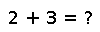# convert

``````..  convert(T, x)

Convert ``x`` to a value of type ``T``.

If ``T`` is an ``Integer`` type, an :exc:`InexactError` will be raised if
``x`` is not representable by ``T``, for example if ``x`` is not
integer-valued, or is outside the range supported by ``T``.

.. doctest::

julia> convert(Int, 3.0)
3

julia> convert(Int, 3.5)
ERROR: InexactError()
in convert at int.jl:209

If ``T`` is a :obj:`AbstractFloat` or :obj:`Rational` type, then it will return
the closest value to ``x`` representable by ``T``.

.. doctest::

julia> x = 1/3
0.3333333333333333

julia> convert(Float32, x)
0.33333334f0

julia> convert(Rational{Int32}, x)
1//3

julia> convert(Rational{Int64}, x)
6004799503160661//18014398509481984``````

## Examples

``````julia> convert(Float64, 4)
4.0

julia> convert(Int8, 'h')
104
julia> convert(Bool, 2)
true
julia> complexNumber = complex(1)
1 + 0im
julia> convert(Int8, complexNumber)
1``````

Checking you are not a robot: